# Improving efficiency in RCTs using propensity scores

Propensity scores have become a popular approach for confounder adjustment in observational studies. The basic idea is to model how the probability of receiving a treatment or exposure depends on the confounders, i.e. the ‘propensity’ to be treated. To estimate the effect of exposure, outcomes are then compared between exposed and unexposed who share the same value of the propensity score. Alternatively the outcome can be regressed on exposure, weighting the observations using the propensity score. For further reading on using propensity scores in observational studies, see for example this nice paper by Peter Austin.

But the topic of this post is on the use of propensity scores in randomized controlled trials. The post was prompted by an excellent seminar recently given by my colleague Elizabeth Williamson, covering the content of her recent paper ‘Variance reduction in randomised trials by inverse probability weighting using the propensity score” (open access paper here).

The first thing to note is that one would not think that propensity scores have a role in RCTs. As noted above, propensity scores are used to adjust for confounding in observational studies. In RCTs, randomization ensures that treatment and other baseline variables are statistically independent, i.e. there is no confounding. So what use are propensity scores here?

The inverse probability of treatment weighting method
In their paper, Williamson, Forbes and White describe how propensity scores can be used to obtain treatment effect estimates with improved efficiency (smaller standard errors). The method is identical to the standard approach, whereby one estimates a propensity score model and then fits the outcome model weighted by the inverse of the propensity score. So, in the first step we fit a model for the binary treatment indicator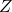with the baseline variables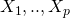as covariates. Again, this may seem quite strange – we know that in truthis just a random Bernoulli variable with probability of ‘success’ equal to one, and that in truth it is not associated with the baseline variables. Ordinarily we would use a logistic regression model to model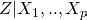.

From the fitted propensity score model, we obtain for each subject in the trial their estimated probability of receiving the treatment (rather than control). Let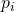denote this fitted probability for subject i. For those who received the treatment (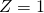) we calculate their weight as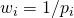while for those who received the control their weight is. We can then obtain our treatment effect estimate by fitting an appropriate outcome model, weighting each subject’s observation by their weight. Note that in this second step we do not include the baseline variables as covariates. For continuous outcomes, we can use linear regression to estimate the mean difference in outcome between treatment groups. For a binary outcome we can fit a logistic or log link regression to estimate the odds ratio or risk ratio.

Williamson et al prove that the resulting estimator is at least as efficient as the estimator which does not make use of the baseline variables. For a continuous outcome which depends linearly on a single baseline variable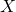, they show that the inverse probability of treatment weighted estimator (IPTW) has the same efficiency as the classical ANCOVA estimator. Thus the propensity score approach achieves an efficiency gain, even though in the RCT setting there is no confounding. The intuition for this result is that in a given trial, a (continuous) baseline variable will always be imbalance between the treatment groups, to some extent. The propensity score / IPTW estimator rebalances the sample so that in the sample data the treatment indicatorand baseline variable are perfectly balanced, which results in a more efficient treatment estimate.

A small simulation study
To the method in action, we can perform a small simulation study with a binary outcome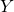and normally distributed baseline variable. We generateusing a logistic regression model. We then estimate the odds ratio with using the baseline variable (the unadjusted analysis), and then implement the IPTW estimator:

```set.seed(68923)

###simulation study
nSim <- 1000
n <- 1000

IPTW_Est<- array(0, dim=nSim)

for (i in 1:nSim) {

z <- 1*(runif(n) < 0.5)
x <- rnorm(n)
xb <- x+z
prob <- exp(xb)/(1+exp(xb))
y <- 1*(runif(n) < prob)

#IPTW estimator
#first we fit the propensity score model
propModel <- glm(z~x, family=binomial)
fitted_p <- fitted(propModel)
#calculate weights
wgt <- 1/fitted_p
wgt[z==0] <- 1/(1-fitted_p[z==0])

iptwMod <- glm(y~z, family=binomial, weight=wgt)
IPTW_Est[i] <- iptwMod\$coef

}
```

We then look at two estimator's performance by looking at their mean and empirical SD across the 1,000 simulations:

```> mean(unadjustedEst)
 0.8392246
 0.1353718
>
> mean(IPTW_Est)
 0.8364911
> sd(IPTW_Est)
 0.1220977
```

We first notice that the mean log odds ratio treatment effect estimates are around 0.84, not the value of 1 used in the data generating mechanism. This is because 0.84 is the marginal odds ratio, whereas 1 is the conditional (on) odds ratio. In the setting of an RCT with binary outcome, where there is no confounding, the marginal effect is always closer to the null (a log odds ratio of zero) than the conditional effect.

Next, we see that the IPTW estimator is less variable in repeated samples than the standard unadjusted estimator. We have thus gained efficiency by using the baseline variable.

One may think that as the size of the trial gets larger and larger, the efficiency advantage of the propensity score method would diminish, since imbalances at baseline will get smaller and smaller in magnitude. However this intuition does not bear out - the IPTW estimator continues to achieve and efficiency gain even as the sample size increases.

Variance estimation
A critical point made by Williamson et al is that the naive standard errors reported at the second stage are conservative, such that the weighting would not appear to result in any efficiency advantage. In this setting it turns out that the effect of estimating the parameters of the propensity score model is to reduce the standard errors of the IPTW estimator. Thus in order to obtain (correctly) narrower standard errors, we must take the first stage step of modelling the propensity score into account.

Williamson et al describe two analytical approaches for doing this. Nonparametric bootstrapping could also be used. This would require wrapping the two steps involved in the IPTW estimator into a small program.

What advantages does the IPTW estimator have over the direct covariate adjustment approach that we would ordinarily use? For continous outcomes, Williamson et al suggest that the IPTW estimator may be preferable over the standard ANCOVA estimator when the standard assumption of linearity made by ANCOVA is false. However, as I have written about previously, ANCOVA is unbiased even when the linearity assumption fails.

For binary outcomes, the IPTW has a number of advantages over more standard approaches. Compared to the unadjusted estimator, we gain efficiency. Furthermore, we will obtain consistent estimates irrespective of how we specify the propensity score model. This follows from the fact that in the case of an RCT, whatever propensity score model we use, it is always correctly specified - the true coefficients are all zero.

A further advantage is that if interest lies in estimating the odds ratio, the adjusted estimator which is obtained by fitting a logistic regression model forwithandas covariate estimates a different parameter, the conditional odds ratio, rather than marginal odds ratio. Of course the former might be of interest in some situations. However, one advantage of the marginal treatment effect is that it is completely unambigious, whereas the conditional effect depends on what baseline variables are adjusted for and the analysis depends on correctly modelling how the outcome depends on the baseline variables.

Williamson et al also note that the IPTW approach may be attractive for estimating risk ratios with improved efficiency. For the log link, the conditional and marginal treatment effects are the same, such that one might use a GLM with log link to estimate the treatment effect with improved efficiency. However, fitting such models is often problematic. In contrast the IPTW estimator does not suffer from convergence problems, yet achieves an efficiency gain.

How to choose the propensity score model
An interesting question is how one should specify the propensity score model in order to achieve the most improvement in efficiency. One might think that this would be achieved by modelling the dependence ofoncorrectly, but of course the correct model fordoes not needin the model! Drawing upon properties of IPTW estimators in observational studies, Williamson et al suggest choosing those variables which are most strongly predictive of outcome in the propensity score model.

In a previous post I've written about an alternative semiparametric approach to extract more efficient treatment effect estimators in RCTs. An advantage of the approach taken there is that the most efficient estimator has been identified, which leads to guidance as to how one can construct an estimator which hopefully achieves optimal or close to optimal efficiency.

### You may also be interested in:

This site uses Akismet to reduce spam. Learn how your comment data is processed.